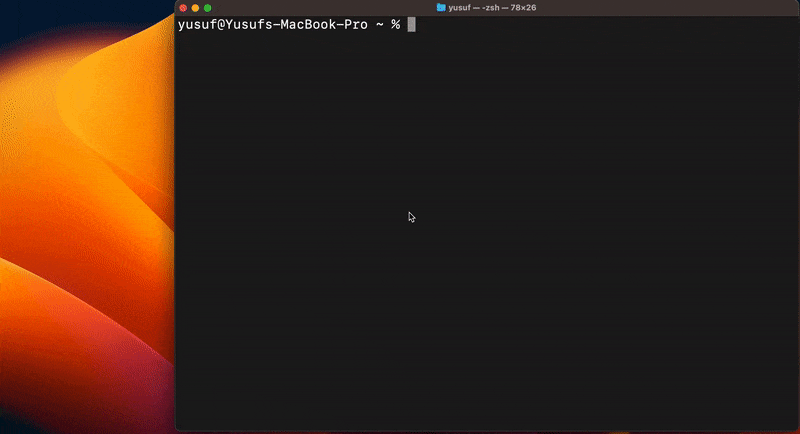# Using the GPU

When making AI predictions, it is often desirable to leverage GPU acceleration to improve performance. In this example, we'll be writing our own Stable Diffusion predictor that leverages the GPU:

## Implementing the Predictor

First, let's make a basic predictor that uses HuggingFace Diffusers to generate an image from a prompt. Create a `stable-diffusion.ipynb` notebook and add the following code cell to install dependencies:

``````# Install dependencies
%pip install torch accelerate transformers diffusers
``````

Next, add a new code cell and paste the following implementation:

``````from diffusers import DiffusionPipeline

pipeline = DiffusionPipeline.from_pretrained("runwayml/stable-diffusion-v1-5")

def predict (prompt: str):
return pipeline(prompt).images
``````

As implemented, the `pipeline` will be executed on the CPU. Next, let's look at how to accelerate it with the GPU:

## Making our Code GPU-Aware

Using the GPU is typically specific to what framework you are using. In our example, we are using PyTorch which means we can use the `Module.to("cuda")` function to move our pipeline to the GPU:

``````from diffusers import DiffusionPipeline

pipeline = DiffusionPipeline.from_pretrained("runwayml/stable-diffusion-v1-5")

def predict (prompt: str):
pipeline.to("cuda")
return pipeline(prompt).images
``````

Note that we added the `to("cuda")` call inside the `predict` function, not outside. This is because the code that exists outside the `predict` function will be run both at provisioning time and at runtime. And the predictor can only have GPU access at runtime, never while provisioning.

Predictors can only have GPU access at runtime, and will never have GPU access while provisioning.

## Creating the Predictor

The final step in using the GPU is to specify the `acceleration` when creating the predictor. Function currently supports the following acceleration types:

`Acceleration`Notes
`CPU`
`A40`
`A100`Short supply. Prefer `A40` where possible.

Let's create our predictor using the `A40` acceleration. Open a terminal and run the following command:

``````# Create the stable diffusion predictor and use the A40 GPU